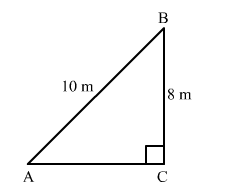# A ladder 10 m long reaches the window of a house 8 m above the ground.

Question:

A ladder 10 m long reaches the window of a house 8 m above the ground. Find the distance of the foot of the ladder from the base of the wall.

Solution:

Let the ladder be AB and BC be the height of the window from the ground.We have:
AB = 10 m and BC = 8 m
Applying Pythagoras theorem in right-angled triangle ACB, we have:

$A B^{2}=A C^{2}+B C^{2}$

$\Rightarrow A C^{2}=A B^{2}-B C^{2}=10^{2}-8^{2}=100-64=36$

$\Rightarrow A C=6 \mathrm{~m}$

Hence, the foot of the ladder is 6 m away from the base of the wall.